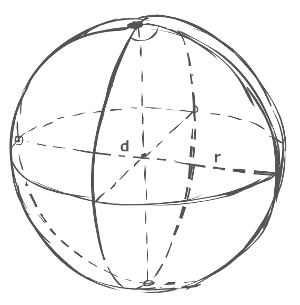# Sphere Calculator

## Definition

Sphere - a three dimensional geometrical figure, the sphere is perfectly round. Similarly to the circle, only in 3 dimensions, it is defined by all points that are in the same distance from any given point. The name comes from the greek word σφαῖρα (sphaira), that means globe or ball.

Equation form:

 d = 2 * r
 r = d 2
 Surface Area (SA) = 4 * π * r²
 Volume (V) = 4 * π * r³ 3